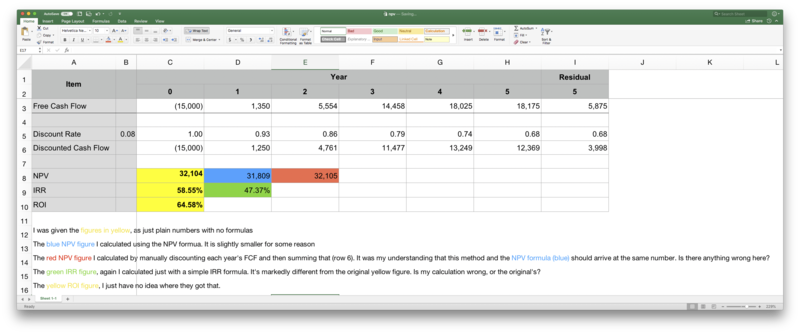https://onedrive.live.com/view.aspx?resid=499D6900D1CAB18C!579&ithint=file%2cxlsx&app=Excel&authkey=!AAJyuIEoSiNYgf4I received an excel document with some plain numbers (i.e. no formulas), and I'm trying to figure out if and how the original calculations are wrong. I've managed to fix all the other errors, but I got stuck trying to determine what (if anything) is wrong with the NPV and IRR calculations.

I have attached both a screenshot & the original excel document.

The original (potentially wrong?) figures are the Yellow cells.

The red NPV figure I calculated by manually discounting each year's FCF and then summing that (row 6). It was my understanding that this method and the NPV formula (blue) should arrive at the same number. Is there anything wrong here?

The green IRR figure, again I calculated just with a simple IRR formula. It's markedly different from the original yellow figure. Is my calculation wrong, or the original's?

The yellow ROI figure, I just have no idea where they got that.